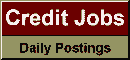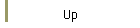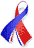DefaultRisk.com the web's biggest credit risk modeling resource.Export citation to:- HTML- Text (plain)- BibTeX- RIS- ReDIF

Portfolio Credit Risk with Extremal Dependence: Asymptotic Analysis and Efficient Simulation

by Achal Bassamboo of Stanford University,
Sandeep Juneja of the Tata Institute of Fundamental Research, and
Assaf Zeevi of Columbia University

February 20, 2006

Abstract: We consider the risk of a portfolio comprised of loans, bonds, and financial instruments that are subject to possible default. In particular, we are interested in performance measures such as the probability that the portfolio incurs large losses over a fixed time horizon and the expected excess loss given that large losses are incurred during this horizon. Contrary to the normal copula that is commonly used in practice (e.g., in the CreditMetrics system), we assume a portfolio dependence structure that is semiparametric, does not hinge solely on correlation, and supports extremal dependence among obligors. A particular instance within the proposed class of models is the so-called t-copula model that is derived from the multivariate Student t distribution and hence generalizes the normal copula model. The size of the portfolio, the heterogenous mix of obligors, and the fact that default events are rare and mutually dependent makes it quite complicated to calculate portfolio credit risk either by means of exact analysis or naïve Monte Carlo simulation. The main contributions of this paper are twofold. We first derive sharp asymptotics for portfolio credit risk that illustrate the implications of extremal dependence among obligors. Using this as a stepping stone, we develop importance sampling algorithms that are shown to be asymptotically optimal and can be used to efficiently compute portfolio credit risk via Monte Carlo simulation.

Keywords: Portfolio, credit, asymptotics, expected shortfall, simulation, importance sampling, rare events, risk management.

Published in: Operations Research, Vol. 56, No. 3, (May-June 2008), pp. 593-606.

Books Referenced in this paper:  (what is this?)